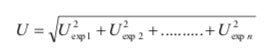Free shipping over €50 - Same day shipping for orders by 4:30pm
Free shipping over €50 - Same day shipping for orders by 4:30pm

# Calibrations

## Traceability and verification

Traceability is an unbroken chain of comparisons, which ensures that each measurement result or value of a standard is linked with a reference to a higher level of accuracy and results in the final, highest level of measurement of the primary standard.

In Greece since 2000, more and more measurements in industrial production have traceability through verification laboratories in national country standards which are accredited to the ISO/IEC 17025 standard -
2005, which are observed by the Hellenic Institute of Metrology . The main means of ensuring the traceability of a measurement is thecalibration of the used measuring instrument, which consists in determining its metrological characteristics. The calibration is therefore the process of checking the accuracy of a measuring instrument (tape measure, caliper, micrometer, scale, etc.). Every metric
system measures some quantity in specific units of measurement according to the SI (Systeme Internationale). Such quantities are time, temperature, mass, length, quantity of a substance, electric current, and luminosity (see Table I). The exact definition of calibration is: "The relevance of results to a standard based on the SI system".

Table 1 . The seven base SI units.

 QUANTITY UNIT SYMBOL Length ( l) meter _ m Mass ( m) kilogram _ kg Time ( t) second _ s Current intensity ( I) ampere _ A Thermodynamic temperature (T) kelvin _ K Shooting ( I) candela _ CD Amount of substance (n) mole _ mol

Table 2 . List of prefixes to SI units.

 International name Symbol Greek Multiply Scale kilo k a thousand 10 3rd _ Thousand Hector h one hundred 10 2 Hundred decade yes ten 10 1 Ten - - - 10 0 =1 Unit deci d tithe 10 -1 Tithe cents c centimeter 10 -2 One hundredth mili m millimeter 10 -3 One thousandth micro m small 10 -6 millionth nano n dwarf 10 -9 billionth

Basic reasons that require the calibration of a measuring instrument:

1. Ensuring that readings from the instrument are consistent with other measurements

2. Determination of the accuracy of the instrument's readings. The security in the indications of the instrument

The consequences of calibration of measuring instruments are the following:

1. The result of the calibration may allow either the adoption of the instrument readings or the correction of the readings

2. Calibration can determine other metrological properties such as the effect of other factors.

3. The result of the calibration is recorded in a document in the form of a calibration certificate or calibration report.

Uncertainty of measurement

More simply, we would say that the calibration is the process of calculating the difference of the values ​​given by the tested instrument from the values ​​of a standard. Consequently, a series of measurements are required to make the calibration. When the calibration is done in an accredited laboratory, it is accompanied by a certificate that lists in detail the measurements that have been made. The same applies when the calibration is done in the laboratory (internal calibration) although these measurements are much less. As we will see, these measurements are always repeated, although they are done at different price levels. In general, from these repeated values, dispersion is calculated in the form of standard deviation (SD : which expresses the amount of change or dispersion of a set of data values) or from the coefficient of variability (CV%: used to compare the variability of samples from different populations . ) but also the difference between the values ​​measured in the instrument and those of the standard. In calibrations the price dispersion comes from these differences. The average difference of these is called uncertainty (Uncertainty or U). The uncertainty (U exp : standard uncertainty) is calculated with the following formulas:

Standard Uncertainty = Mean Instrument Value – Standard Value

The standard uncertainty has the same units as the instrument. But when the uncertainty is used in further calculations, the relative uncertainty is used instead:

Relative uncertainty = (Difference between instrument and standard values)/ (theoretical value)

The relative uncertainty relates the standard uncertainty to the magnitude of the theoretical value.

The uncertainty we now consider in the lab is the expanded uncertainty (U) which is twice the standard for a 95% confidence interval. That is , U = 2 U exp In the event that an experiment involves more than one instrument that has been verified and their uncertainty has been calculated, then the expanded uncertainty is equal to the sum of all individual standard uncertainties.